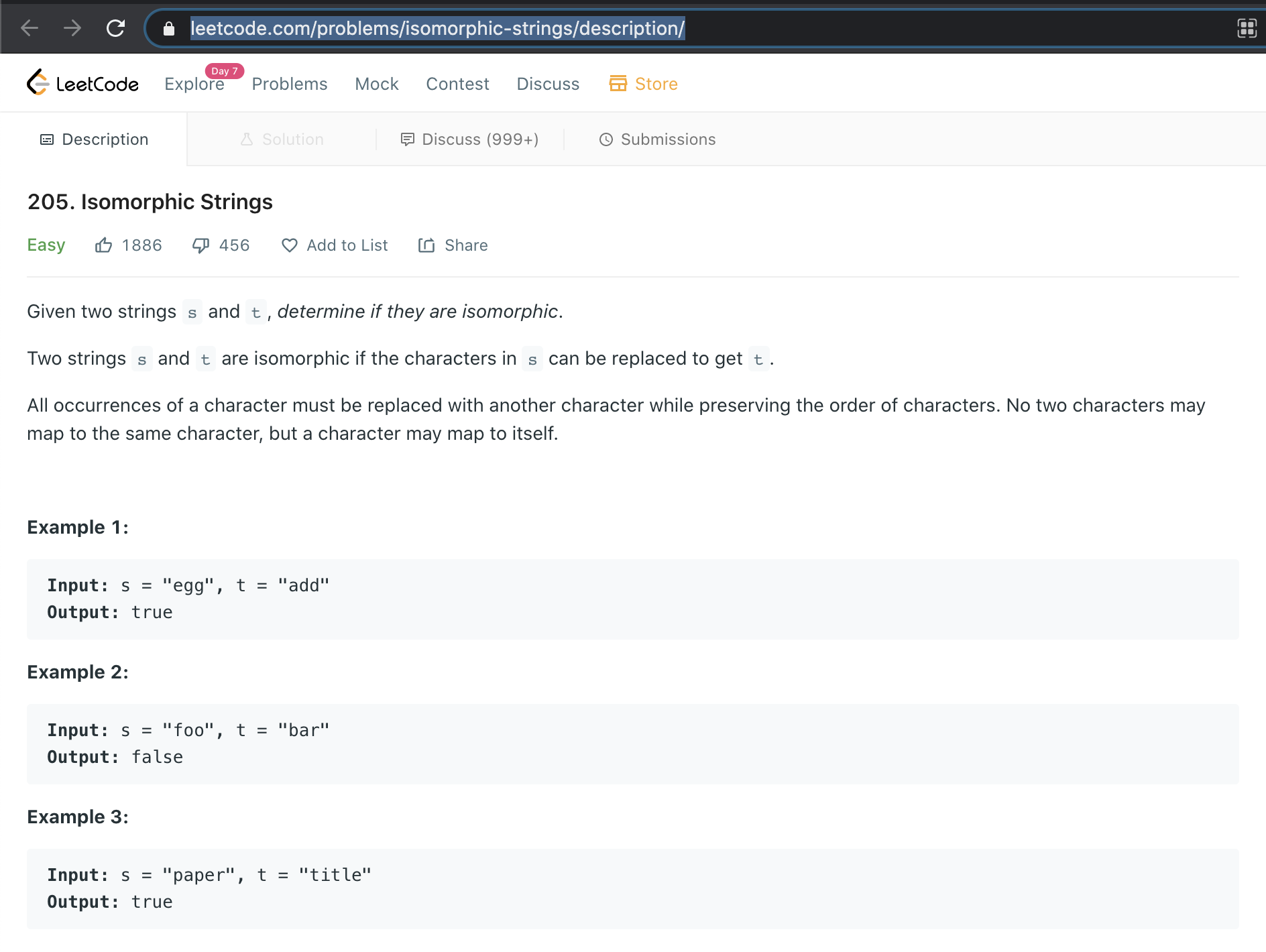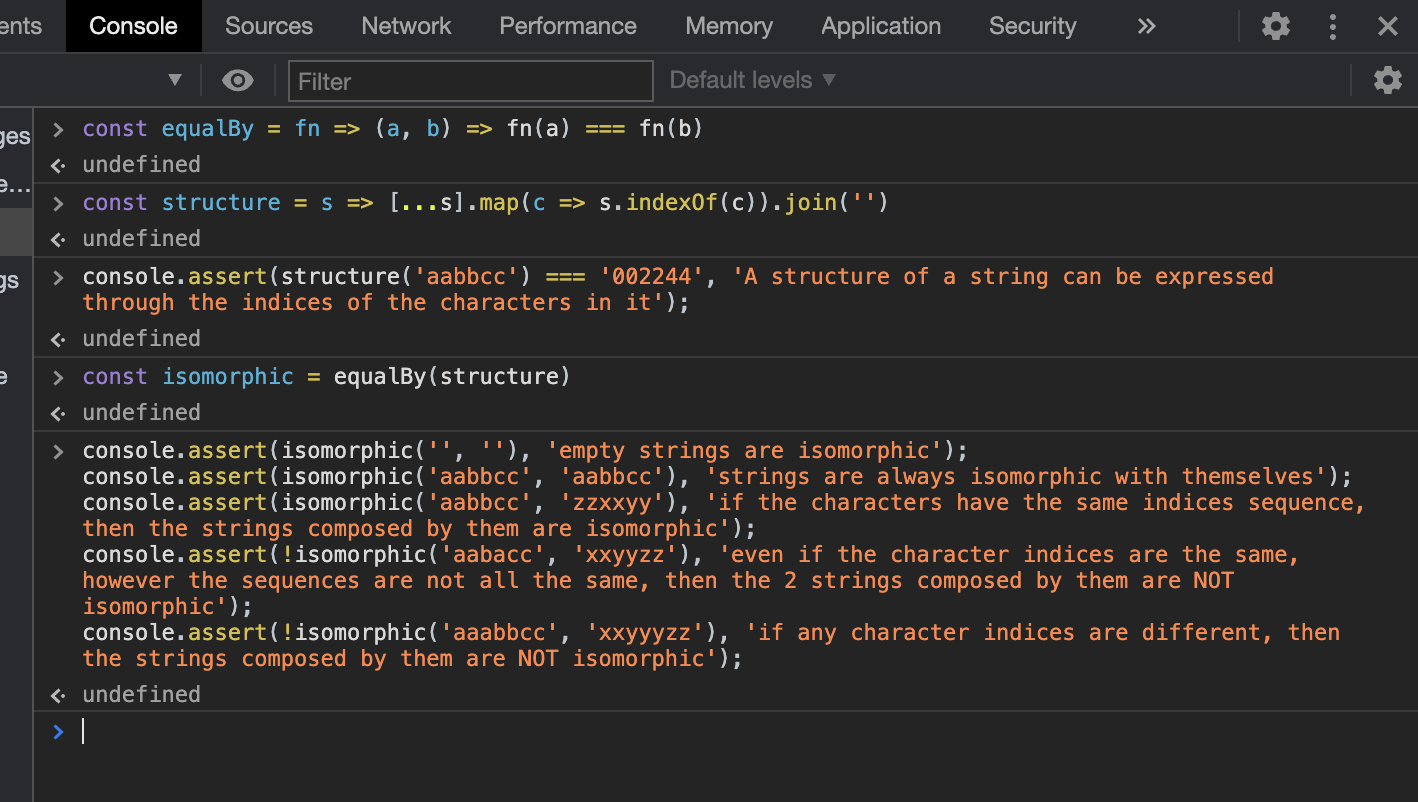This post will present a look and feel about functional style programming, gives you a glance about what it would look like if we wrote programs in a functional programming way.

It's not a real strict functional programming guide, it just shows how interesting yet powerful if we handle problems in a functional programming mind.

## Problem

It's quite common challenge to ask you implement an algorithm to detect if 2 strings are isomorphic during a programming job interview, there might be many answers on it. Let's do it again.

## Tools

• A browser. So we can write pure JavaScript to implement it by pressing F12 with a running brower at hand.By analyzing the requirement, we can see actually the term `isomorphic` reflects the requirement quite well, which means having the same form, in other words, the forms (or structures) in a way are the same(equal). So we can just write some code to express the meanings:

``````const isomorphic = equalBy(structure)
``````

So far we have the signature of the function `equalBy` , let's implement it:

``````const equalBy = fn => (a, b) => fn(a) === fn(b)
``````

It's natural and self expressed.

Now we take a closer look into `isomorphic` , we found it cares only the structure of the string, and doesn't give a shit to the detail characters in it. So how to we express the form (or structure) of the string?

By examining the examples given in the requirement we come up with an idea to express the structure of a string by the character indices in the string, which can be expressed by numbers so it abstracts from the detail characters. So we write the following code:

``````const structure = s => [...s].map(c => s.indexOf(c)).join('-')
``````

This line of code is a little bit long, let's test it and document it:

``````console.assert(structure('aabbcc') === '002244', 'A structure of a string can be expressed through the indices of the characters in it');
``````

By far we have both `equalBy` and `structure` , so `isomorphic` is ready to run! We can write some tests to show it:

``````console.assert(isomorphic('', ''), 'empty strings are isomorphic');
console.assert(isomorphic('aabbcc', 'aabbcc'), 'strings are always isomorphic with themselves');
console.assert(isomorphic('aabbcc', 'zzxxyy'), 'if the characters have the same indices sequence, then the strings composed by them are isomorphic');
console.assert(!isomorphic('aabacc', 'xxyyzz'), 'even if the character indices are the same, however the sequences are not all the same, then the 2 strings composed by them are NOT isomorphic');
console.assert(!isomorphic('aaabbcc', 'xxyyyzz'), 'if any character indices are different, then the strings composed by them are NOT isomorphic');
console.assert(!isomorphic('abcdefghijk', 'abcdefghijba'), 'if the lengths are different, then the strings are NOT isomorphic');
``````

We ran the tests, all pass!## Summary

So the implementation code for `isomorphic` is only 3 lines in total:

``````const equalBy = fn => (a, b) => fn(a) === fn(b)

const structure = s => [...s].map(c => s.indexOf(c)).join('-')

const isomorphic = equalBy(structure)
``````

You can see it's a `pointless` way of writing code, besides cool, it solves problem elegantly even to a simple extend!

You can try on your browser or check it in leetcode too: https://leetcode.com/submissions/detail/465004270/ https://leetcode.com/submissions/detail/530009145/

This post is also available on DEV.